# Notes on Multiobjective Optimization: Quality Assessment of Pareto Set Approximations

## Quantifying Quality General Considerations

A general optimization problem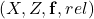: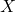denotes the decision space,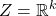is the objective space,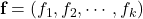is the vector of objective functions, and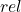represents a binary relation over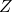that defines a partial order of the objective space, which in turn induces a preorder of the decision space.

Weak Pareto dominance: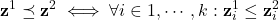Pareto dominance: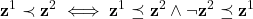A corresponding set problem: the symbol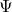stands for the sets of all Pareto set approximations over, the set of all Pareto front approximations overis represented by,,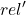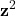Quality Indicators: give more precise statements that quantify the difference in quality on a continuous scale, every quality indicator represents certain assumptions about the decision maker’s preferences, binary quality indicators exist.

## Properties of Unary Quality Indicators

### Monotonicitystrict: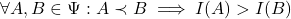### Scaling

invariance: indicator values remain the same after after a strictly monotonic transformation

independent: indicator values remain same order after after a strictly monotonic transformation

### Computation effort

runtime complexity depending on the number of solutions and the number of objectives

known Pareto optimal set? reference point or set?

## Discussion of Selected Unary Quality Indicators

### Terminology: a Pareto set approximation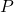: the Pareto-optimal front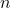: the number of objectives

### Outer Diameter

to be maximized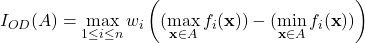### Proportion of Pareto-Optimal Objective Vectors Found

to be maximized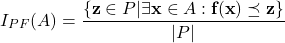### Cardinality

to be maximized

The number of elements of the set

### Hypervolume Indicator

to be maximized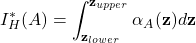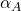is the attainment function### Completeness Indicator

to be maximized### Epsilon Indicator Family

to be minimizedmultiplicative-dominance relation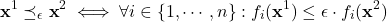additive-dominance relation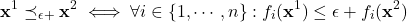unary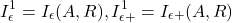### The D Indicator Family

to be minimized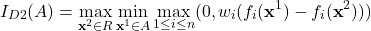### The R Indicator Family

to be minimized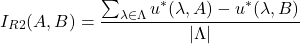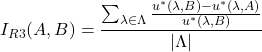unary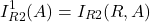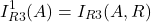usual utility functions: weighted linear function, the weighted Tchebycheff function, the augmented Tchebycheff function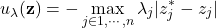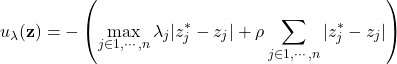where### Uniformity Measures

the standard deviation of nearest neighbor distances

## Indicator Combinations and Binary Indicators

e.g. combine the epsilon indicator and the hypervolume indicator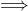strictly monotonic, much less expensive

## Stochasticity: General Considerations

transform the sample of approximation sets into

• a sample of indicator values: first transforms the samples of Pareto set approximations into samples of real values using quality indicators; then, the resulting samples of indicator values are compared based on standard statistical testing procedures
• an empirical attainment function: counts the number of approximation sets by which each objective vector is attained and normalizes the resulting number with the overall sample size
• a sample of ranks: for each Pareto set approximation generated by one optimizer it is computed by how many Pareto set approximations produced by another optimizer it is dominated

## Sample Transformations

### Dominance Ranking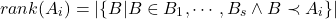• recommended to be the first step in any comparison
• quality indicators or the attainment function are optional

### Quality Indicators

the same pros and cons as aforementioned

### Empirical Attainment Function

for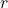runs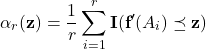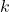%-attainment set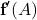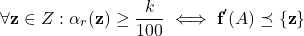attainment surface of• less information is lost
• computationally expensive

## Statistical Testing

### Fundamentals

subject: a sample of Pareto set approximations generated from multiple runs of an optimizer

• descriptive statistics: mean and variance(box plots)
• statistical inferences: by testing the possibility that the null hypothesis is true(statistical tests)

#### Statistical Inferences

null hypothesis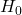: “samplesand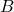are drawn from the same distribution” or “samplesandare drawn from distributions with the same mean value”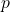-value: the probability of obtaining a finding at least as ‘impressive’ as that obtained, computed by an inferential statistical test: the significance level defines the largest acceptable p-value and represents a threshold that is user-defined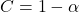: confidence level-valuealternative hypothesis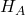at a significance level of: “samplecomes from a better distribution than sample” given by a one-tailed test or “sample A and sample B are from different distributions” given by a two-tailed test

#### Non-parametric Statistical Inference: Rank and Permutation Tests

nonparametric tests is better than parametric tests(assuming known distribution)

• rank tests: less powerful, less sensitive to outliers and computationally cheap
• permutation tests: more powerful, better for cases with many tied values, expensive

### Comparing Samples of Dominance Ranks

A test statistic: sum over the ranks in each of the two samples and take the difference of these sums

whether the value of the test statistic is significant? the set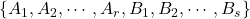is partitioned into one set ofapproximations and another set of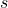approximations; for each partitioning the difference between the rank sums can be determined, finally yielding a distribution of rank sum differences

### Comparing Sample Indicators Values

2 optimizers: the Mann-Whitney rank sum test or Fisher’s permutation test

more than 2 optimizers: the Kruskal-Wallis test

### Comparing Empirical Attainment Functions

the Kolmogorov-Smirnov (KS) test: measures the maximum difference between the ECDFs and assesses the statistical significance of this difference

Which optimizer is better in which area? plot the difference in frequencies of attaining the goal or the-value of the difference

#### Matched Samples

matched: the influence of one or more random variables is partially removed from consideration

statistical tests to use: the Wilcoxon signed rank test, Fisher’s matched samples test

#### Multiple Testing

problem: the-values are not reliable when

• There are more than two algorithms and we wish to make inferences about performance differences between all or a subset of them
• There are just two algorithms, but we wish to make multiple statistical tests of their performance, e.g., considering more than one indicator

solution

• Minimize the number of different tests carried out on a pair of algorithms by carefully choosing which tests to apply before collecting the data. Collect independent data for each test to be carried out
• Apply the tests on the same data but use methods for correcting the-values for the reduction in confidence associated with data reuse, e.g. the significance level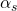for each distinct test is set to(Bonferroni correction)

#### Assessing Worst-Case or Best-Case Performance

use permutation methods

## Summary

• dominance-ranking
• quality indicators(optional, reflect preference)
• empirical attainment functions(optional, next level detail)

## Reference

Branke, J., Branke, J., Deb, K., Miettinen, K., & Slowiński, R. (Eds.). (2008). Multiobjective optimization: Interactive and evolutionary approaches (Vol. 5252). Springer Science & Business Media.

### 1人评论了“Notes on Multiobjective Optimization: Quality Assessment of Pareto Set Approximations”

1. Pingback: MOOP开发日志 - Fivyex's Blog